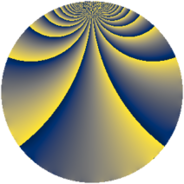Properties

 Label 1620.1.vLevel $1620$ Weight $1$ Character orbit 1620.v Rep. character $\chi_{1620}(217,\cdot)$ Character field $\Q(\zeta_{12})$ Dimension $8$ Newform subspaces $2$ Sturm bound $324$ Trace bound $11$

Related objects

Defining parameters

 Level: $$N$$ $$=$$ $$1620 = 2^{2} \cdot 3^{4} \cdot 5$$ Weight: $$k$$ $$=$$ $$1$$ Character orbit: $$[\chi]$$ $$=$$ 1620.v (of order $$12$$ and degree $$4$$) Character conductor: $$\operatorname{cond}(\chi)$$ $$=$$ $$45$$ Character field: $$\Q(\zeta_{12})$$ Newform subspaces: $$2$$ Sturm bound: $$324$$ Trace bound: $$11$$

Dimensions

The following table gives the dimensions of various subspaces of $$M_{1}(1620, [\chi])$$.

Total New Old
Modular forms 152 8 144
Cusp forms 8 8 0
Eisenstein series 144 0 144

The following table gives the dimensions of subspaces with specified projective image type.

$$D_n$$ $$A_4$$ $$S_4$$ $$A_5$$
Dimension 0 0 8 0

Trace form

 $$8 q + 4 q^{7} + O(q^{10})$$ $$8 q + 4 q^{7} + 4 q^{25} - 4 q^{31} + 4 q^{67} + 8 q^{73} + 4 q^{85} + O(q^{100})$$

Decomposition of $$S_{1}^{\mathrm{new}}(1620, [\chi])$$ into newform subspaces

Label Dim. $$A$$ Field Image CM RM Traces $q$-expansion
$a_{2}$ $a_{3}$ $a_{5}$ $a_{7}$
1620.1.v.a $4$ $0.808$ $$\Q(\zeta_{12})$$ $S_{4}$ None None $$0$$ $$0$$ $$0$$ $$2$$ $$q+\zeta_{12}^{5}q^{5}+(-\zeta_{12}-\zeta_{12}^{4})q^{7}+\zeta_{12}^{4}q^{11}+\cdots$$
1620.1.v.b $4$ $0.808$ $$\Q(\zeta_{12})$$ $S_{4}$ None None $$0$$ $$0$$ $$0$$ $$2$$ $$q-\zeta_{12}^{5}q^{5}+(-\zeta_{12}-\zeta_{12}^{4})q^{7}-\zeta_{12}^{4}q^{11}+\cdots$$